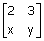Related Articles
GATE | GATE CS 2010 | Question 29
• Last Updated : 14 Feb, 2018

Consider the following matrix
A =If the eigenvalues of A are 4 and 8, then

(A) x=4, y=10
(B) x=5, y=8
(C) x=-3, y=9
(D) x= -4, y=10

Explanation: